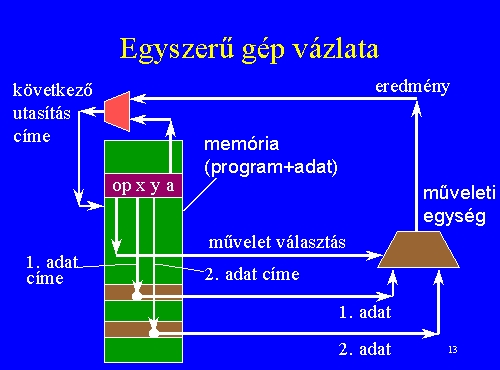Istenes Zoltán - Computer Architectures - Lecture notes

introduction, basic concepts
What is a computer ?
Utilisation of the computers
What are the parts of a computer ?
Peripheriques connected to a computer
data, information
processing
Difference between the computer and the calculator

Structure of a simple machineHardware - software
program execution

arithmetic and logical unit
bus
register
control unit

instruction types
• data transfer instructions
• arithmetic and logical instructions
• program control instructions
• I/O instructions
• system control instructions

number representations
binary system
number conversions (base 2 <-> base 10)
positive integer numbers
signed integer numbers
sign magnitude representation
1's complement representation
2's complement representation
excess-N representation
fixed point representation
normalisation
floating point representation (IEEE 764 standard)
ASCII

construction of a ALU
logical gates
logical circuits

Istenes Zoltán

2000 oct. 16.